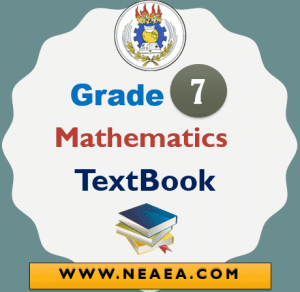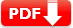# Ethiopian Grade 7 Mathematics Textbook [PDF]

Ethiopian Grade 7 Mathematics Textbook [PDF]: The Grade 7 Mathematics Student Textbook is provided by the Ethiopian Ministry Of Education (MOE) for free.

According to the new Curriculum Framework for primary education (Grades 7), the Ethiopian Grade 7 Mathematics Textbook has been prepared considering international best practices. The principles will serve as guides in the subsequent development of curriculum materials across all grade levels.## Ethiopian Grade 7 Mathematics Textbook

 Country Ethiopia Publisher MoE, Ethiopia Class Grade 7 Subject Mathematics Type Textbook Purpose Student Text Book Date of Uploaded June 15, 2020 Total Units 5 TextBook Version 1.0

### Unit :1 Rational Numbers

In the previous grades, you had already learned about fractions and decimals. These numbers together with integers from a bigger set of numbers known as the set of rational numbers. In this unit, you will learn about rational numbers and their basic properties. You will also learn how to perform the four fundamental operations on rational numbers.

After completing this unit, you should be able to:
 define and represent rational numbers as fractions.
 show the relationship among, and.
 order rational numbers.
 Perform operations with rational numbers.

 Download Unit 1 in PDF### Unit:2 Rational Numbers

Introduction Based on your knowledge of working with variables and solving one step of linear equations and inequalities. You will learn more about solving linear equations and inequalities involving more than one step. When you do this you will apply the rules of equivalent transformations of equations and inequalities appropriately.

After completing this unit, you should be able to:
 solve linear equations using transformation rules.
 solve linear inequalities using transformation rules.

 Download Unit 2 in PDF### Unit:3 Ratio and Proportion

You may see when people are comparing two or more quantities that are measured in the same unit. Have you ever compared such quantities by yourself or with your friends? In this topic, you will learn the mathematical concept of comparing quantities known as the ratio, proportion, percentage, and the application of percentage to calculate profit, loss, and interest

After completing this unit, you should be able to:
 understand the notions of ratio and proportions.
 solve problems related to percentage.
 make use of the concept of percentage to solve problems of profit, loss, and simple interest.

 Download Unit 3 in PDF### Unit:4 Data Handling

The collection of data from a group of things helps us to understand more about these things in the group. To do this they collected data should be presented systematically or pictorially so as to analyze them. In this unit, you will learn how to collect simple data and present them pictorially and do some calculations on them to study their nature or property.

After completing this unit, you should be able to:
 collect data and construct simple line graphs and pie charts for a given data.
 calculate the mean, median, and mode of a given data.
 find the rage of a given data.

 Download Unit 4 in PDF### Unit:5 Geometric Figures and Measurement

In this unit, you will extend your knowledge of geometric figures. You will exercise how to construct quadrilaterals and describe their properties using your construction. You will also learn more about triangles. Moreover, you will be able to calculate the areas and perimeter of Plane figures including solid figures like surface areas and volumes of prisms and circular cylinders.

After completing this unit, you should be able to:
 identify, construct and describe properties of quadrilaterals such as trapezium and parallelogram.
 identify the difference between convex and concave polygons.
 find the sum of the measures of the interior angles of a convex polygon.
 calculate perimeters and areas of triangles and trapeziums.

 Download Unit 5 in PDF